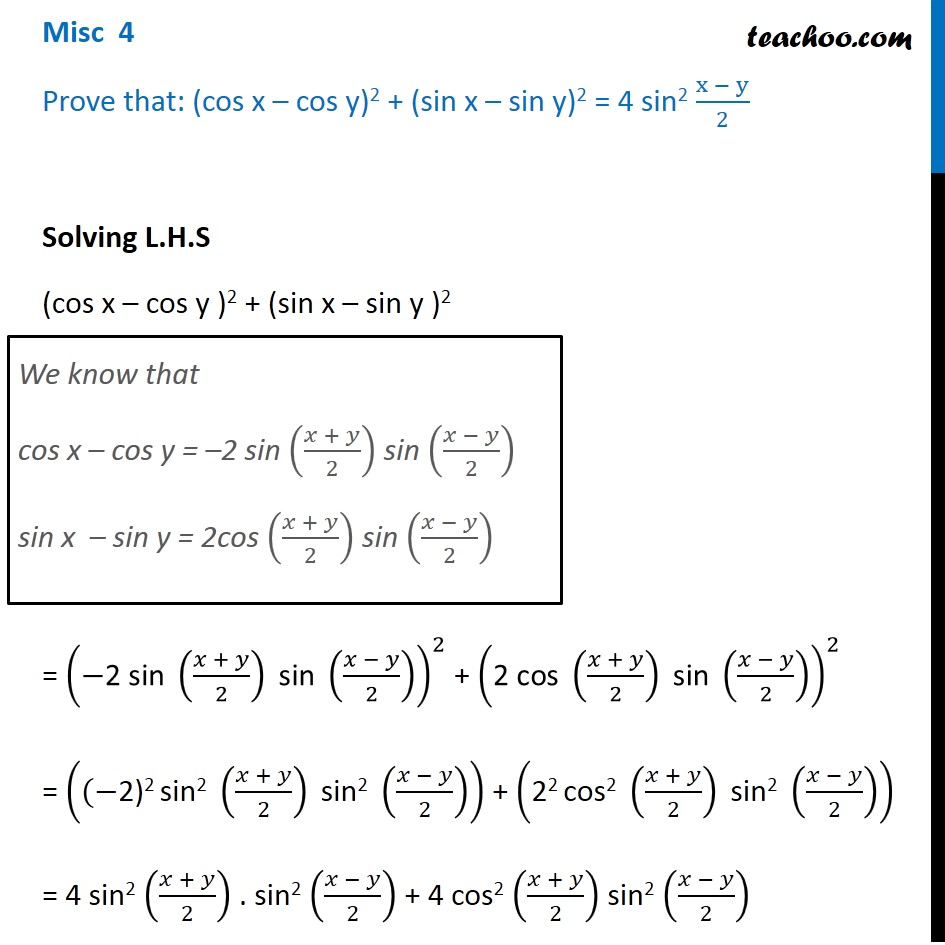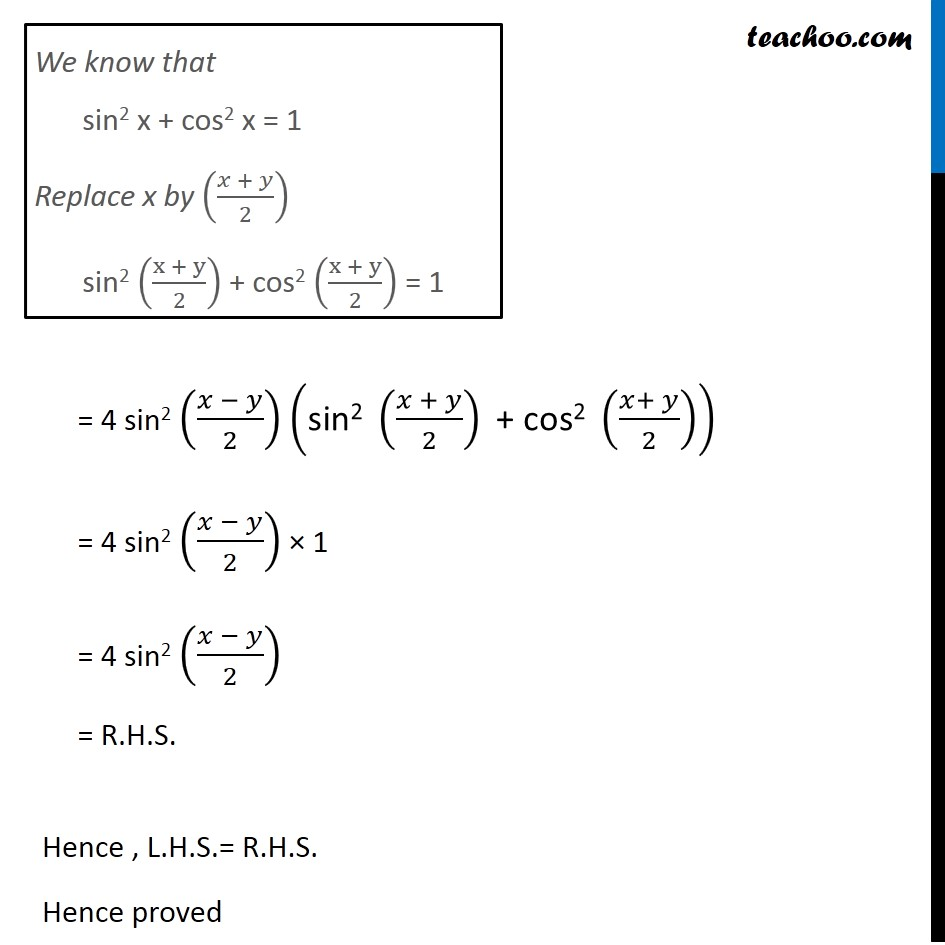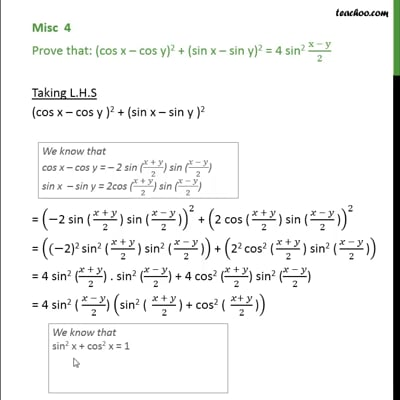Miscellaneous

Chapter 3 Class 11 Trigonometric Functions
Serial order wiseThis video is only available for Teachoo black users

Solve all your doubts with Teachoo Black (new monthly pack available now!)

### Transcript

Misc 4 Prove that: (cos x – cos y)2 + (sin x – sin y)2 = 4 sin2 (x − y)/2 Solving L.H.S (cos x – cos y )2 + (sin x – sin y )2 = (−"2 sin " ((𝑥 + 𝑦)/2)" sin " ((𝑥 − 𝑦)/2))^2 + ("2 cos " ((𝑥 + 𝑦)/2)" sin " ((𝑥 − 𝑦)/2))^2 = ((−"2)2 sin2 " ((𝑥 + 𝑦)/2)" sin2 " ((𝑥 − 𝑦)/2)) + ("22 cos2 " ((𝑥 + 𝑦)/2)" sin2 " ((𝑥 − 𝑦)/2)) = 4 sin2 ((𝑥 + 𝑦)/2) . sin2 ((𝑥 − 𝑦)/2) + 4 cos2 ((𝑥 + 𝑦)/2) sin2 ((𝑥 − 𝑦)/2) We know that cos x – cos y = –2 sin ((𝑥 + 𝑦)/2) sin ((𝑥 − 𝑦)/2) sin x – sin y = 2cos ((𝑥 + 𝑦)/2) sin ((𝑥 − 𝑦)/2) We know that sin2 x + cos2 x = 1 Replace x by ((𝑥 + 𝑦)/2) sin2 ((x + y)/2) + cos2 ((x + y)/2) = 1 = 4 sin2 ((𝑥 − 𝑦)/2) ("sin2 " ((𝑥 + 𝑦)/2)" + cos2 " ((𝑥+ 𝑦)/2)) = 4 sin2 ((𝑥 − 𝑦)/2) × 1 = 4 sin2 ((𝑥 − 𝑦)/2) = R.H.S. Hence , L.H.S.= R.H.S. Hence proved